#### Lagrangian

##### Description

Lagrangian[oqu] gives the unpolarized quark operator. Lagrangian[oqp] gives the polarized quark operator. Lagrangian["QCD"] gives part of the QCD Lagrangian.

##### Examples
``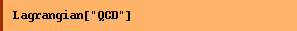``
`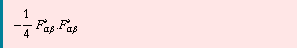`

Twist-2 operator product expansion operators

``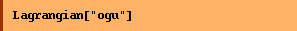``
`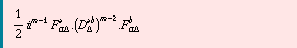`
``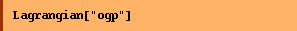``
`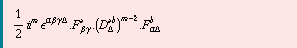`
``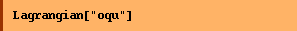``
`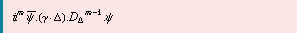`
``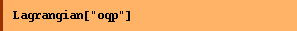``
`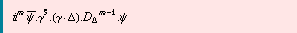`

Converted from the Mathematica notebook Lagrangian.nb